# RD Sharma Solutions For Class 12 Maths Exercise 4.10 Chapter 4 Inverse Trigonometric Functions

Get the detailed RD Sharma Solutions for Class 12 Maths Exercise 4.10 Chapter 4 Inverse Trigonometric Functions, which is available here. From the exam point of view, subject experts have prepared the solutions in a step by step format in accordance with the CBSE syllabus. Students gain knowledge and increase their confidence by following the steps, when practised regularly. This exercise explains the topic of properties of inverse trigonometric functions. To know more about the construction of graphs using trigonometric functions students can download RD Sharma Solutions for Class 12 Maths.

## Download the PDF of RD Sharma Solutions For Class 12 Chapter 4 – Inverse Trigonometric Functions Exercise 4.10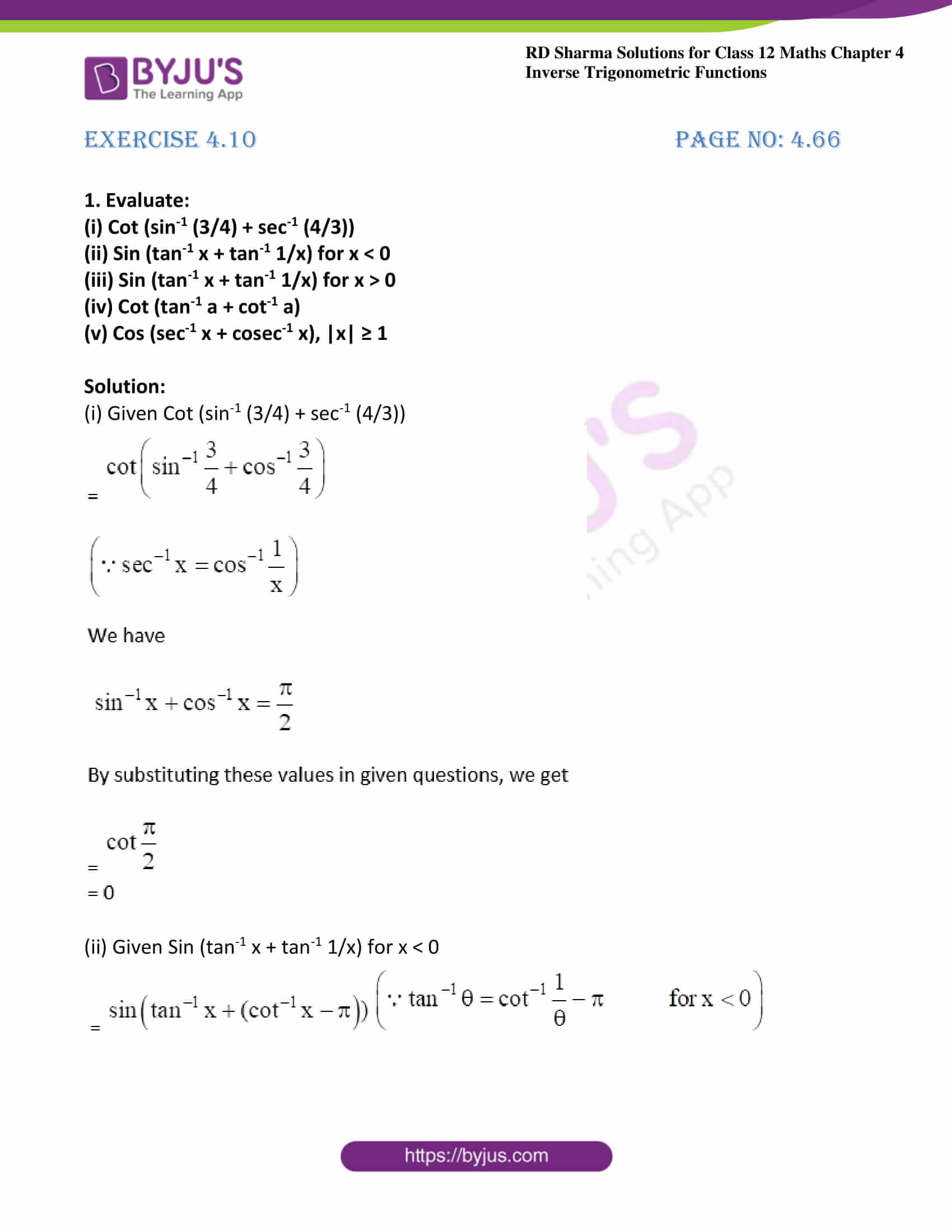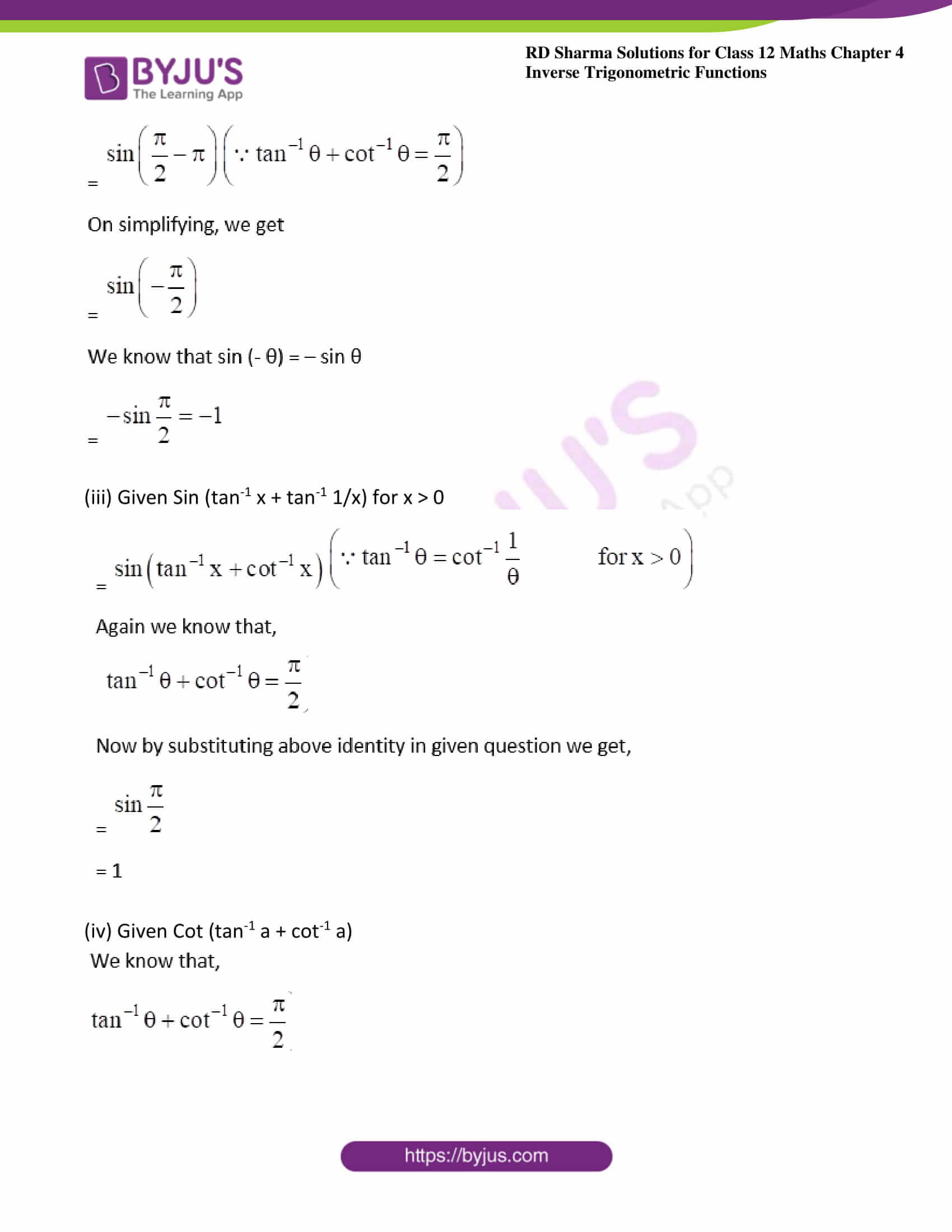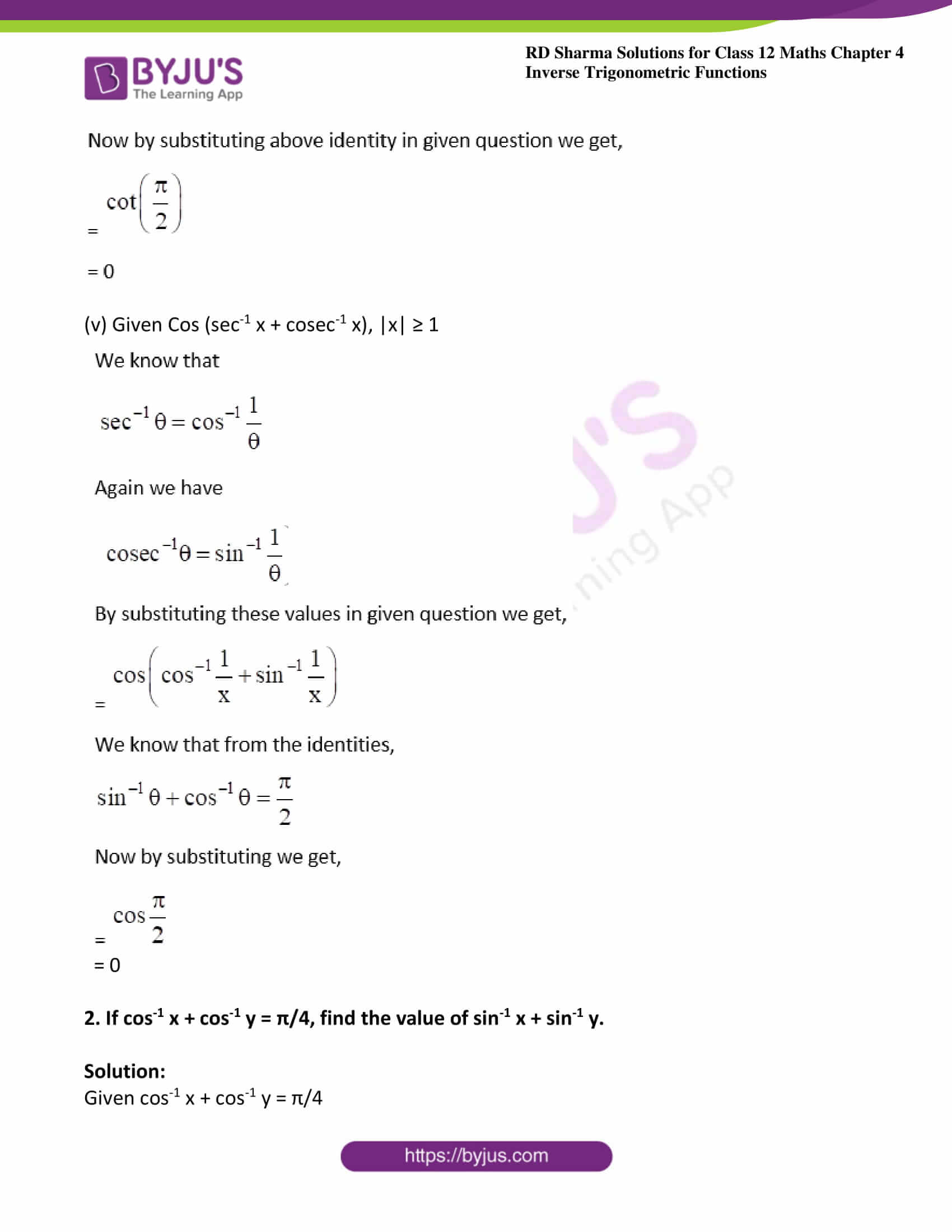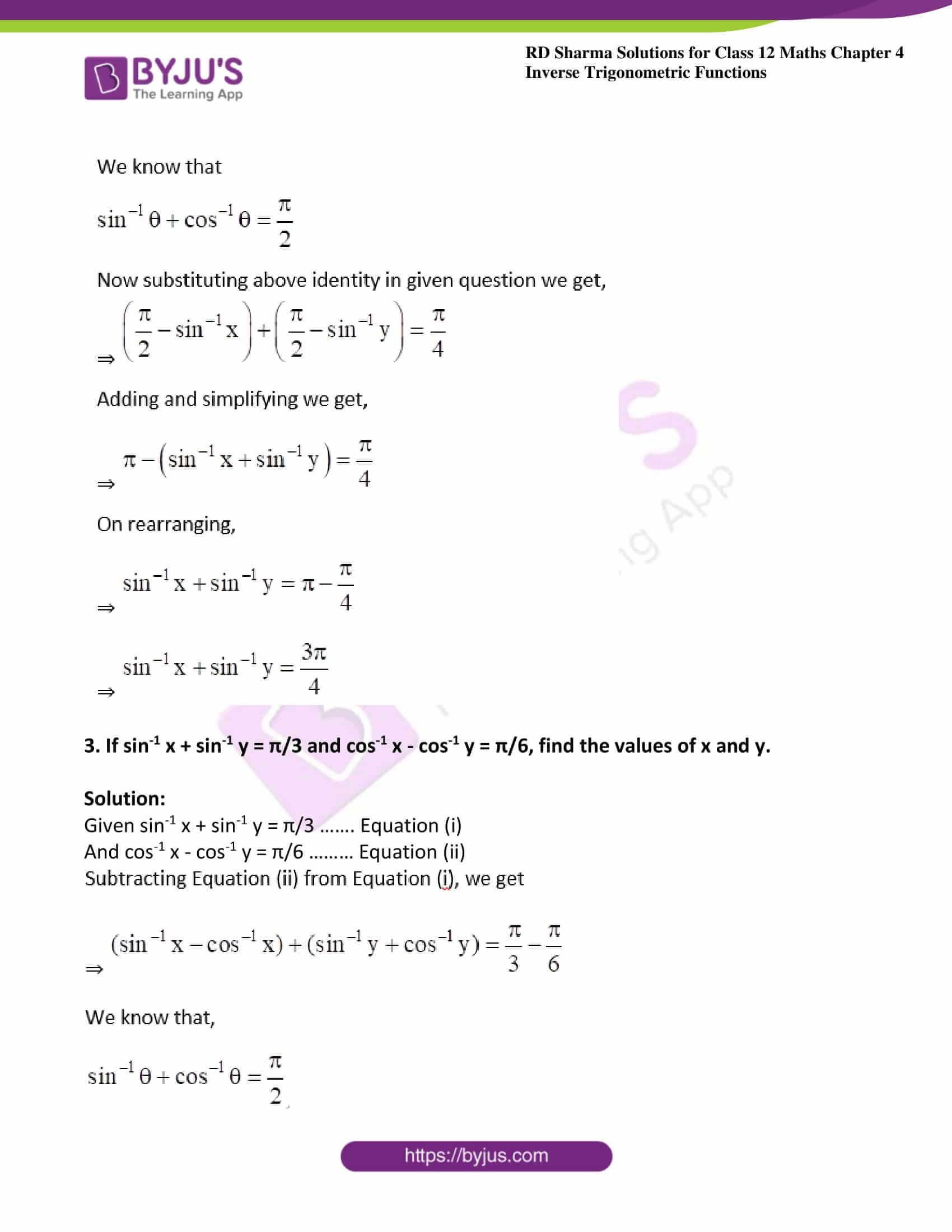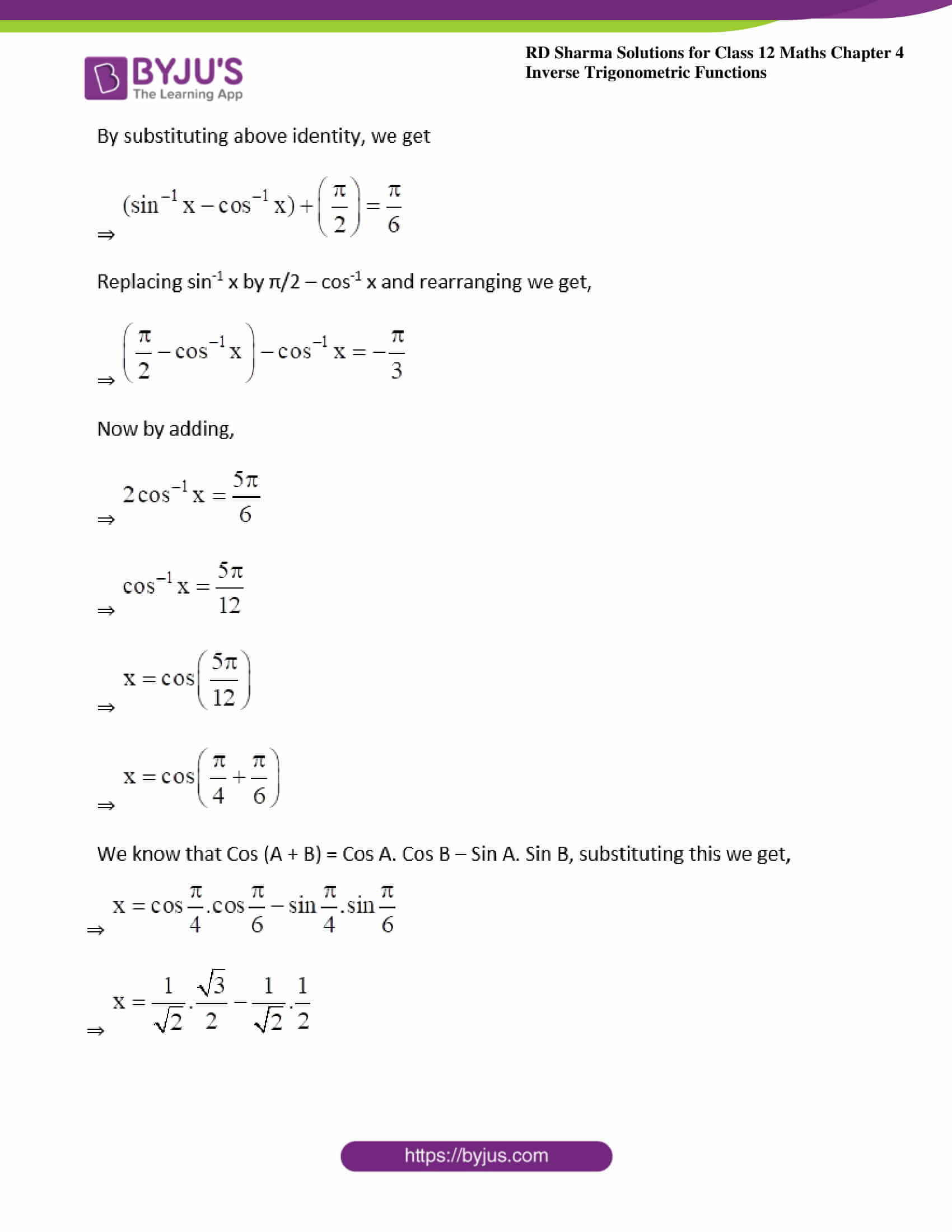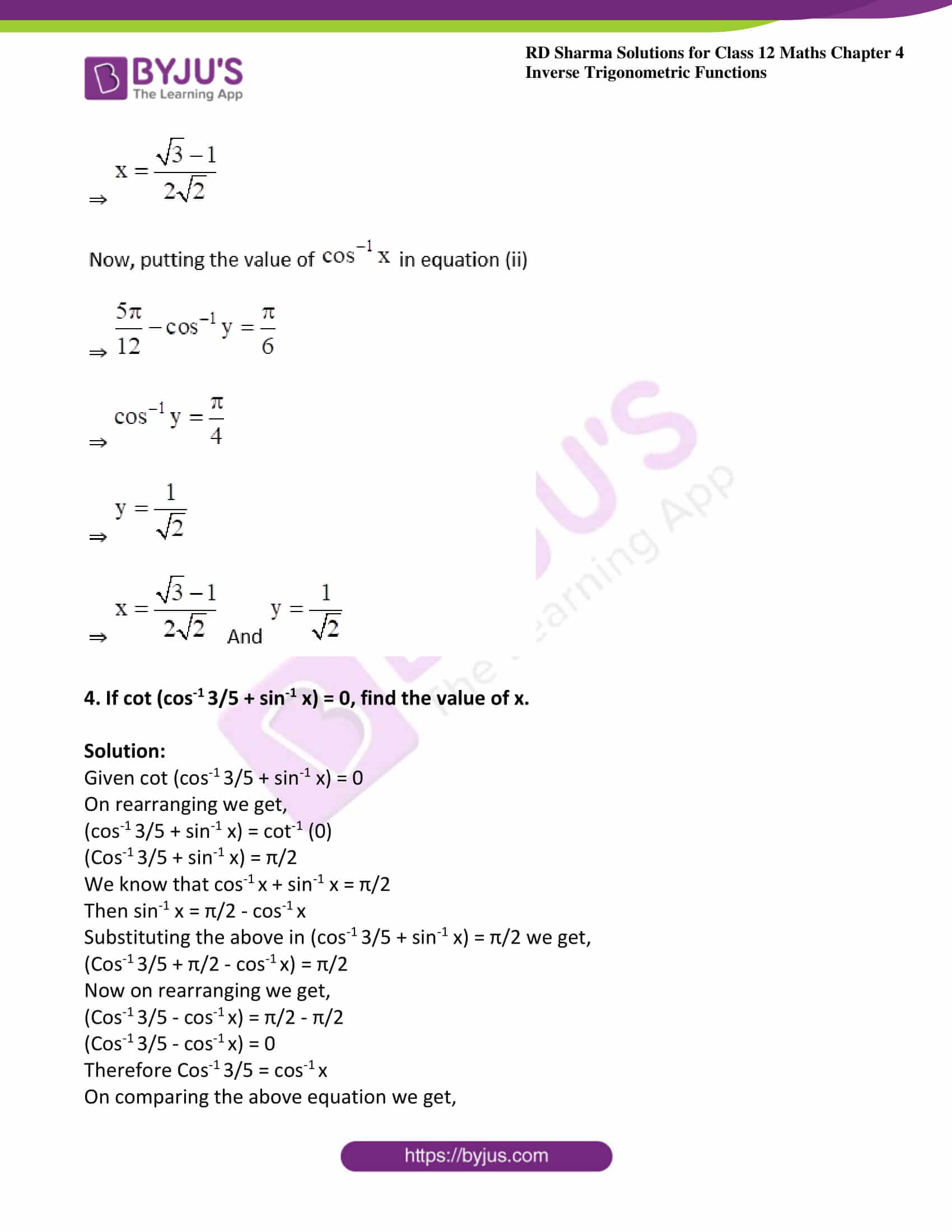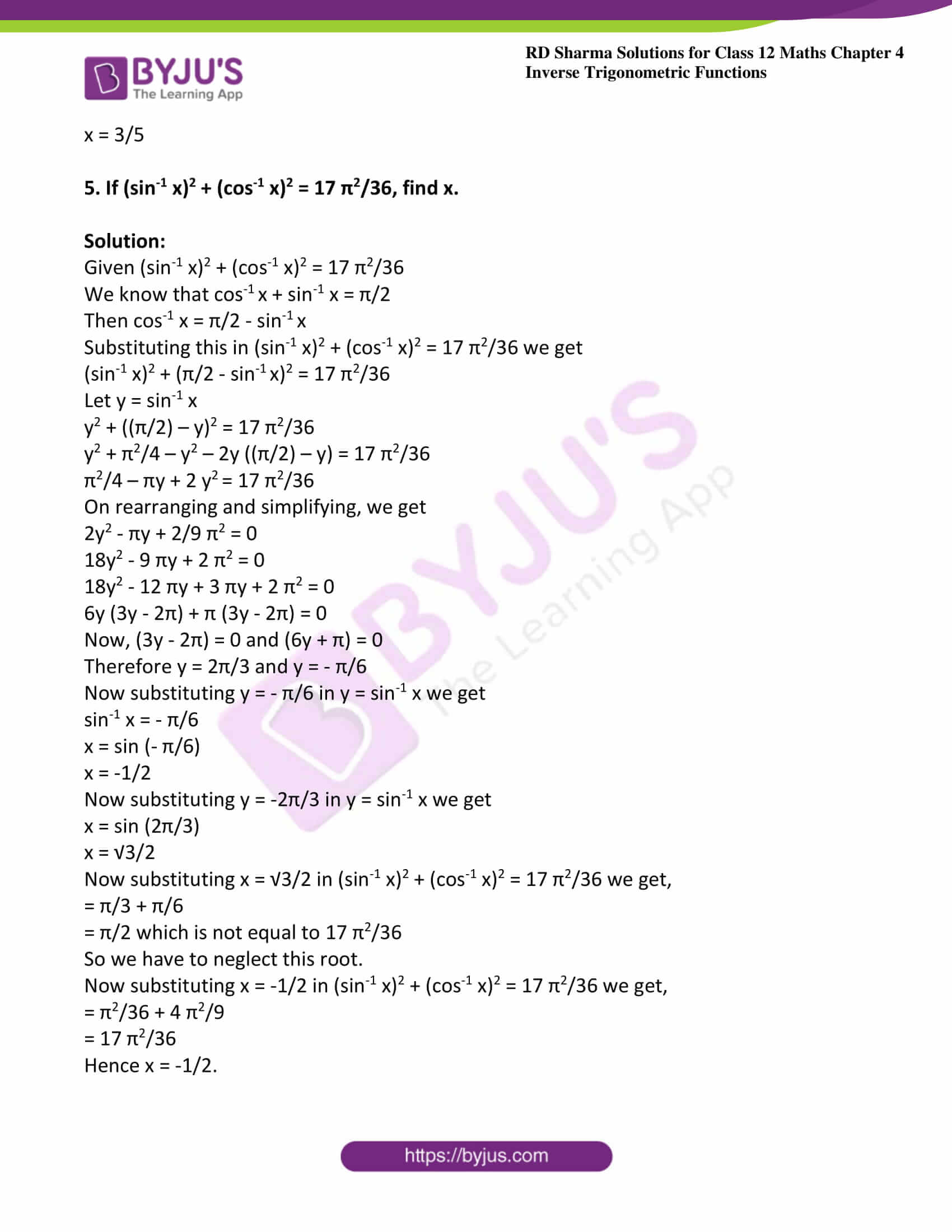### Exercise 4.10 Page No: 4.66

1. Evaluate:

(i) Cot (sin-1 (3/4) + sec-1 (4/3))

(ii) Sin (tan-1 x + tan-1 1/x) for x < 0

(iii) Sin (tan-1 x + tan-1 1/x) for x > 0

(iv) Cot (tan-1 a + cot-1 a)

(v) Cos (sec-1 x + cosec-1 x), |x| ≥ 1

Solution:

(i) Given Cot (sin-1 (3/4) + sec-1 (4/3))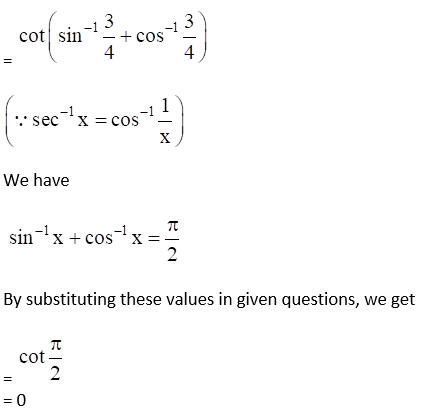(ii) Given Sin (tan-1 x + tan-1 1/x) for x < 0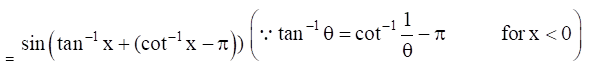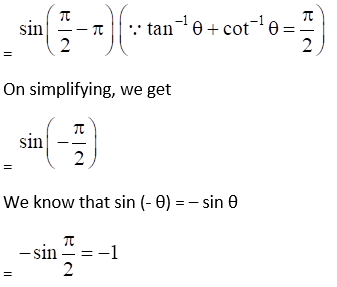(iii) Given Sin (tan-1 x + tan-1 1/x) for x > 0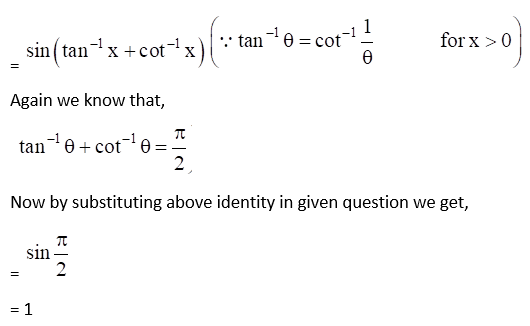(iv) Given Cot (tan-1 a + cot-1 a)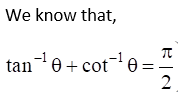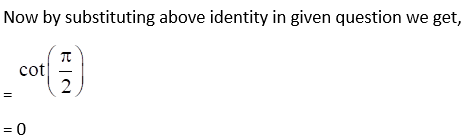(v) Given Cos (sec-1 x + cosec-1 x), |x| ≥ 1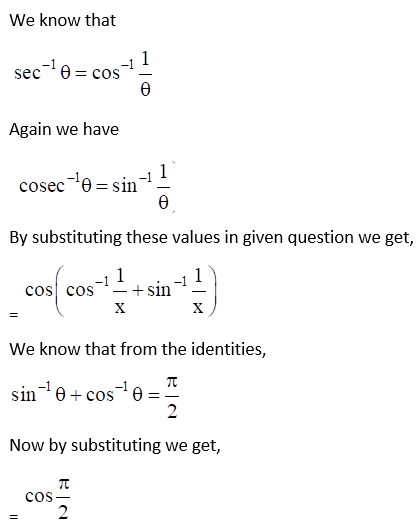= 0

2. If cos-1 x + cos-1 y = π/4, find the value of sin-1 x + sin-1 y.

Solution:

Given cos-1 x + cos-1 y = π/4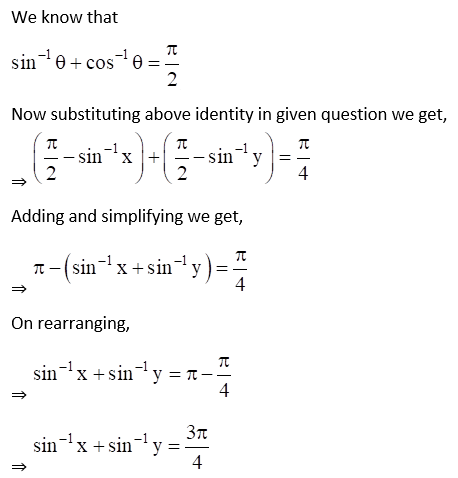3. If sin-1 x + sin-1 y = π/3 and cos-1 x – cos-1 y = π/6, find the values of x and y.

Solution:

Given sin-1 x + sin-1 y = π/3 ……. Equation (i)

And cos-1 x – cos-1 y = π/6 ……… Equation (ii)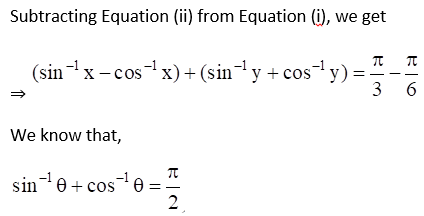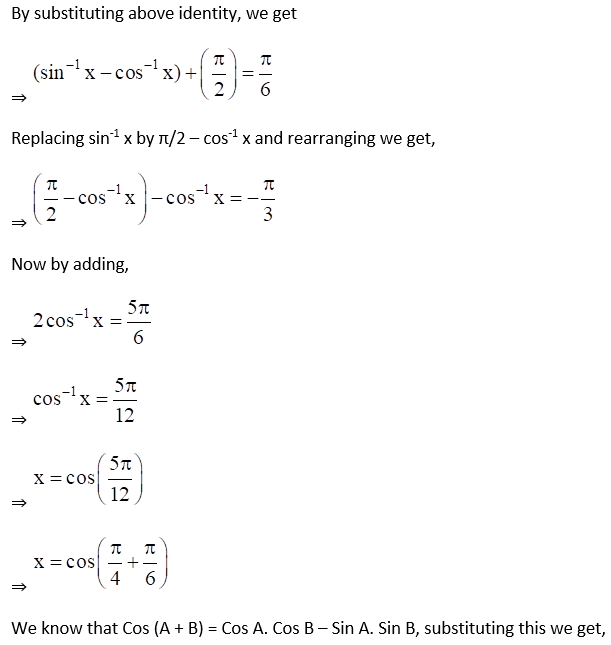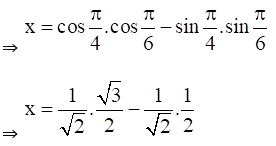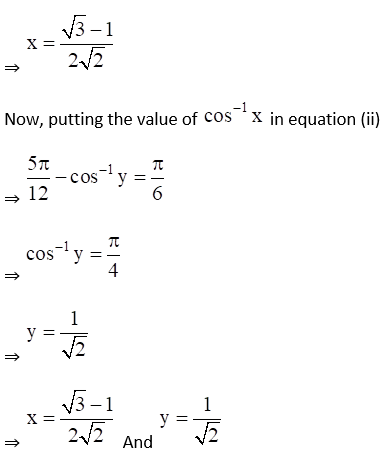4. If cot (cos-1 3/5 + sin-1 x) = 0, find the value of x.

Solution:

Given cot (cos-1 3/5 + sin-1 x) = 0

On rearranging we get,

(cos-1 3/5 + sin-1 x) = cot-1 (0)

(Cos-1 3/5 + sin-1 x) = π/2

We know that cos-1 x + sin-1 x = π/2

Then sin-1 x = π/2 – cos-1 x

Substituting the above in (cos-1 3/5 + sin-1 x) = π/2 we get,

(Cos-1 3/5 + π/2 – cos-1 x) = π/2

Now on rearranging we get,

(Cos-1 3/5 – cos-1 x) = π/2 – π/2

(Cos-1 3/5 – cos-1 x) = 0

Therefore Cos-1 3/5 = cos-1 x

On comparing the above equation we get,

x = 3/5

5. If (sin-1 x)2 + (cos-1 x)2 = 17 π2/36, find x.

Solution:

Given (sin-1 x)2 + (cos-1 x)2 = 17 π2/36

We know that cos-1 x + sin-1 x = π/2

Then cos-1 x = π/2 – sin-1 x

Substituting this in (sin-1 x)2 + (cos-1 x)2 = 17 π2/36 we get

(sin-1 x)2 + (π/2 – sin-1 x)2 = 17 π2/36

Let y = sin-1 x

y2 + ((π/2) – y)2 = 17 π2/36

y2 + π2/4 – y2 – 2y ((π/2) – y) = 17 π2/36

π2/4 – πy + 2 y2 = 17 π2/36

On rearranging and simplifying, we get

2y2 – πy + 2/9 π2 = 0

18y2 – 9 πy + 2 π2 = 0

18y2 – 12 πy + 3 πy + 2 π2 = 0

6y (3y – 2π) + π (3y – 2π) = 0

Now, (3y – 2π) = 0 and (6y + π) = 0

Therefore y = 2π/3 and y = – π/6

Now substituting y = – π/6 in y = sin-1 x we get

sin-1 x = – π/6

x = sin (- π/6)

x = -1/2

Now substituting y = -2π/3 in y = sin-1 x we get

x = sin (2π/3)

x = √3/2

Now substituting x = √3/2 in (sin-1 x)2 + (cos-1 x)2 = 17 π2/36 we get,

= π/3 + π/6

= π/2 which is not equal to 17 π2/36

So we have to neglect this root.

Now substituting x = -1/2 in (sin-1 x)2 + (cos-1 x)2 = 17 π2/36 we get,

= π2/36 + 4 π2/9

= 17 π2/36

Hence x = -1/2.

### Access other exercises of RD Sharma Solutions For Class 12 Chapter 4 – Inverse Trigonometric Functions

Exercise 4.1 Solutions

Exercise 4.2 Solutions

Exercise 4.3 Solutions

Exercise 4.4 Solutions

Exercise 4.5 Solutions

Exercise 4.6 Solutions

Exercise 4.7 Solutions

Exercise 4.8 Solutions

Exercise 4.9 Solutions

Exercise 4.11 Solutions

Exercise 4.12 Solutions

Exercise 4.13 Solutions

Exercise 4.14 Solutions